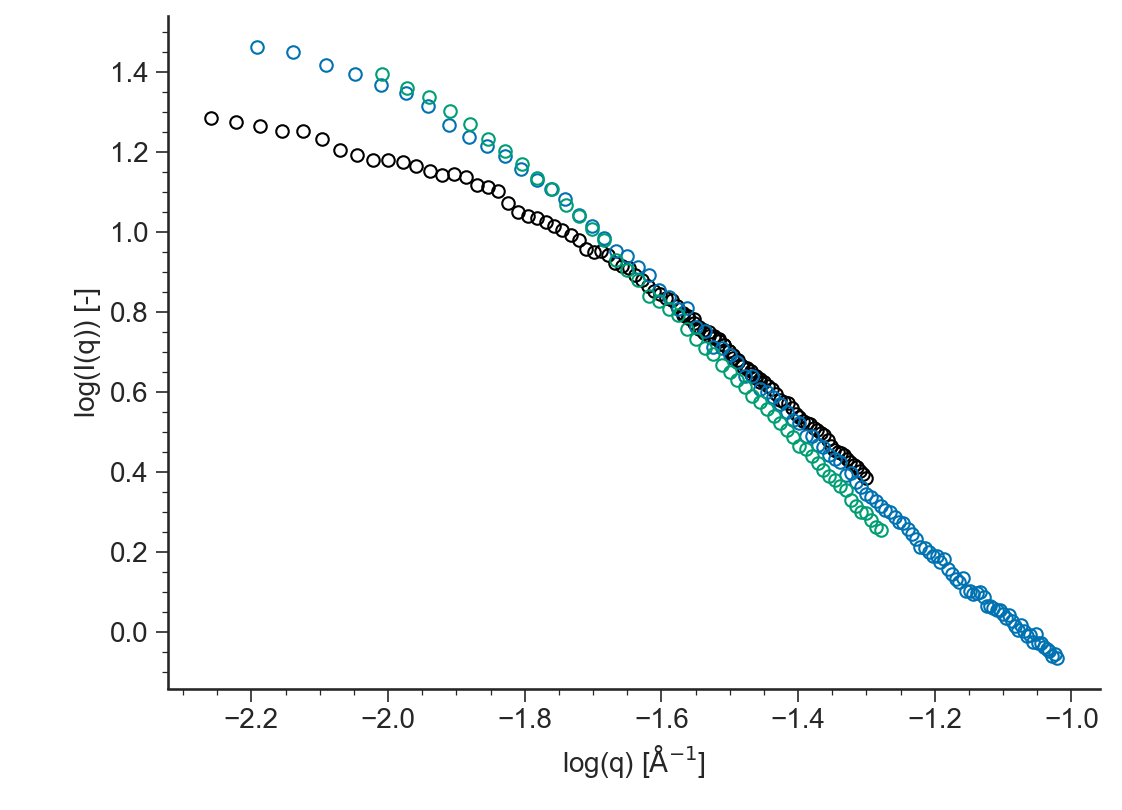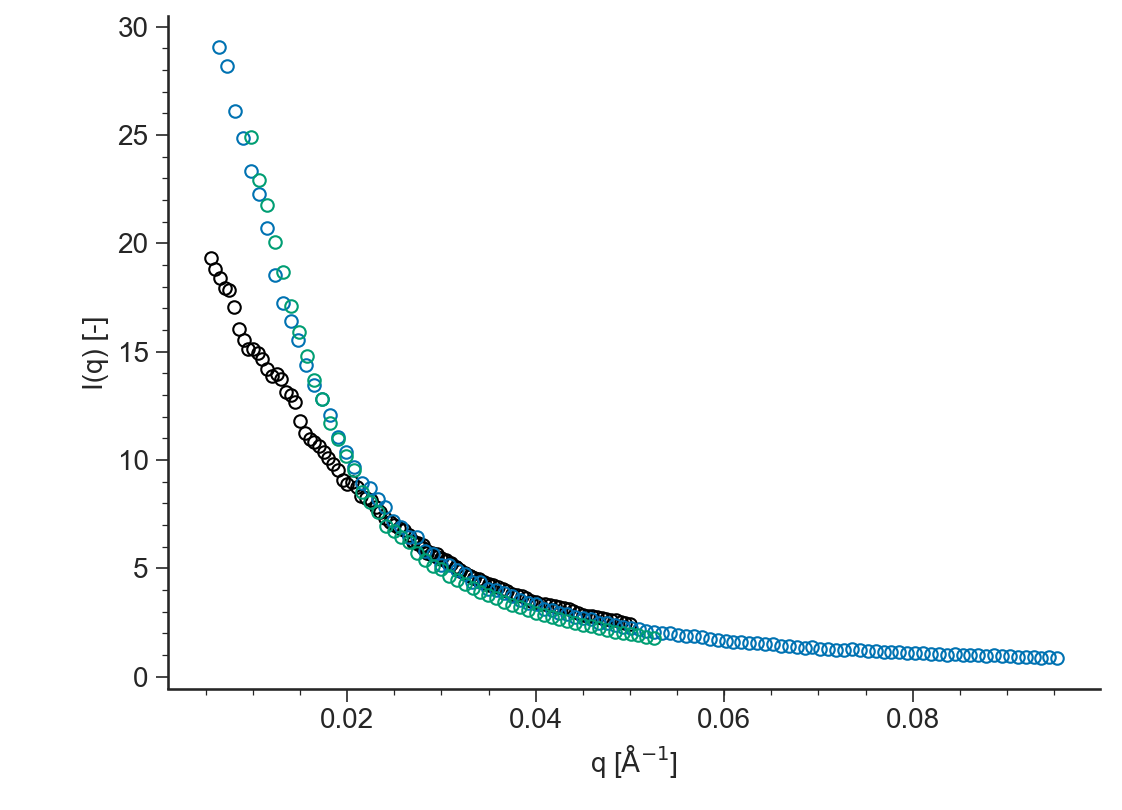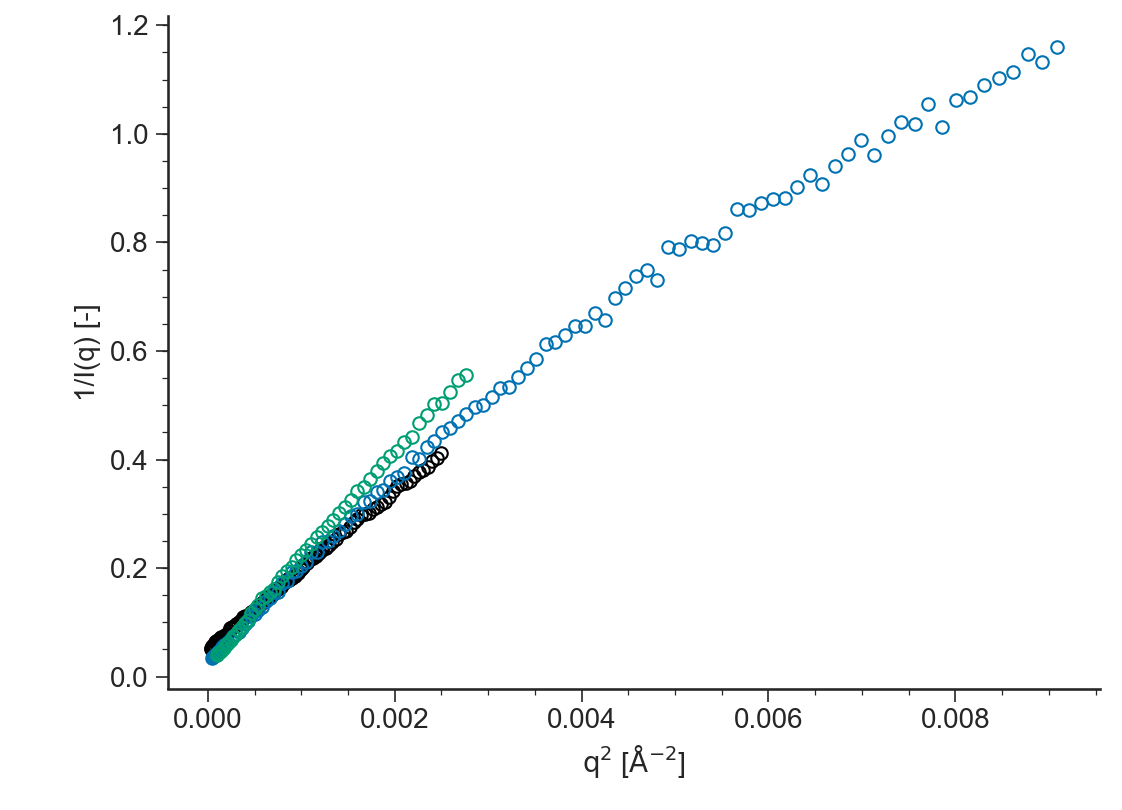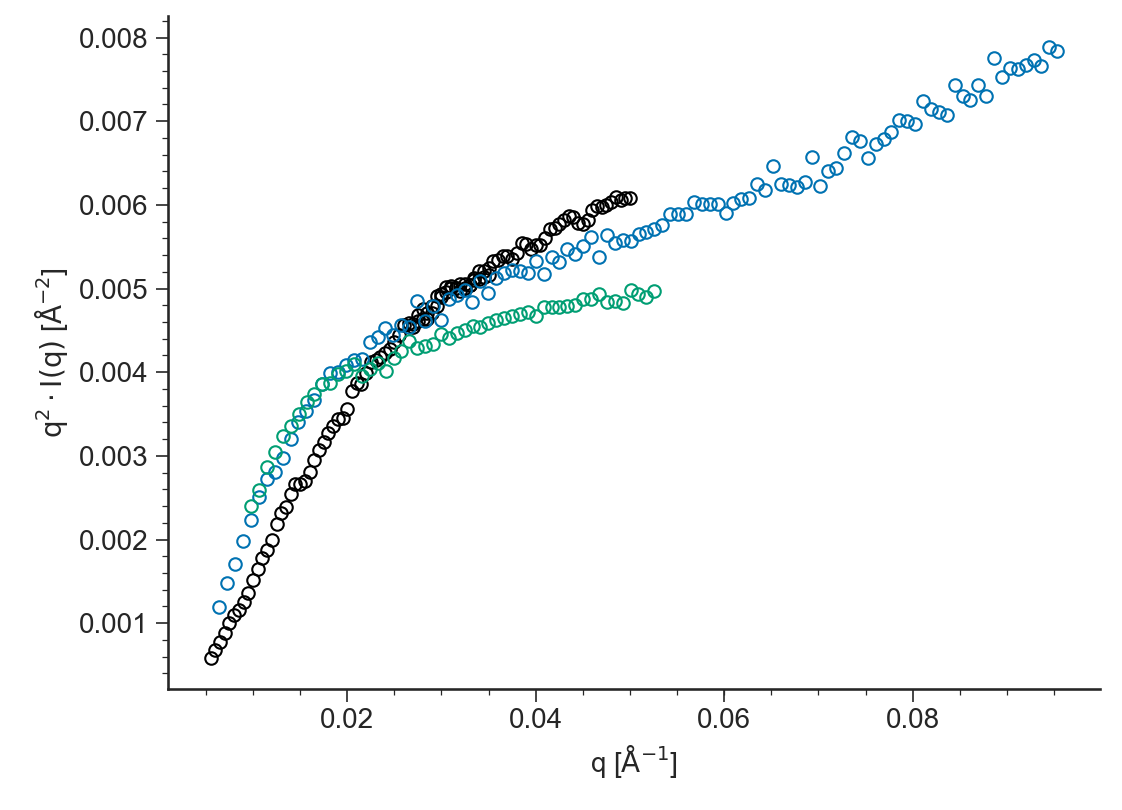# SANS: General description¶

Contents

## Purpose¶

Application to Analyze Data from SANS experiments

## Data Files¶

• The first line of the file should contain the sample parameters separated by semi-colons (;). It may contain any number of parameters which will be read and saved as file-parameter in RepTate.

• Then the data columns should appear, separated by spaces or tabs.

### .sans extension¶

Text files with .sans extension should be organised as follows:

• .sans files should contaion at least the parameter values for the:

1. Sample molecular weight Mw,

2. Volume fraction of deuterated chains Phi.

• 2 columns separated by spaces or tabs containing respectively:

1. scattering vector, $$q$$,

2. scattered intensity, $$I(q)$$,

A correct .sans file looks like:

Mw=407;Phi=0.964;
0.0098083         24.9121
0.010647          22.9176
0.011486          21.7702
0.012325          20.0757
0.013164          18.6836
...               ...


## Views¶

### log(I(q))¶

BaseApplicationSANS.viewLogSANS()[source]

Logarithm of the scattered intensity $$\log (I(q))$$ vs the logarithm of the scattering vector $$\log(q)$$### I(q)¶

BaseApplicationSANS.viewSANS()[source]

Scattered intensity $$I(q)$$ vs scattering vector $$q$$ (both axes in logarithmic scale)### Zimm¶

BaseApplicationSANS.viewZimm()[source]

Zimm plot: $$I(q)^{-1}$$ vs $$q^2$$### Kratky¶

BaseApplicationSANS.viewKratky()[source]

Kratky plot: $$q^2\cdot I(q)$$ vs $$q$$